# Class 8 NCERT Solutions – Chapter 15 Introduction to Graphs – Exercise 15.1

• Last Updated : 24 Nov, 2020

### Question 1: The following graph shows the temperature of a patient in a hospital, recorded every hour.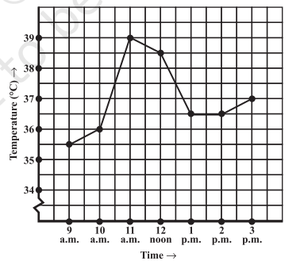(i) What was the patient’s temperature at 1 p.m.?

Solution:

The patient’s temperature at 1 p.m. was 36.5oC.

(ii) When was the patient’s temperature 38.5° C?

Solution:

The patient’s temperature was 38.5oC at 12 noon.

(iii) The patient’s temperature was the same two times during the period given. What were these two times?

Solution:

Patient’s temperature at 2 p.m. was the same as the patient’s temperature at 1 p.m. (36.5oC).

(iv) What was the temperature at 1.30 p.m.? How did you arrive at your answer?

Solution:

Patient’s temperature was 36.5oC at 1:30 p.m.
The line in between 1 p.m. and 2 p.m. represents 1:30 p.m. And the line in between 36oC and 37oC
represents 36.5oC. Therefore the temperature at 1:30 p.m. was 36.5oC.

(v) During which periods did the patients’ temperature showed an upward trend?

Solution:

Patient’s temperature showed an upward trend between 9 a.m. and 11 a.m. and also the patient’s
temperature showed an upward trend between 2 p.m. and 3 p.m. for the second time.

### Question 2: The following line graph shows the yearly sales figures for a manufacturing company.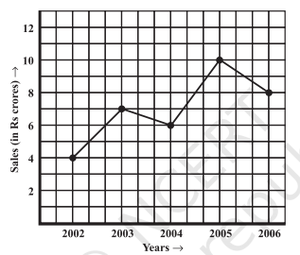(a) What were the sales in (i) 2002 (ii) 2006?

Solution:

(i) Sales in 2002 was 4 crore rupees.

(ii) Sales in 2006 was 8 crore rupees.

(b) What were the sales in (i) 2003 (ii) 2005?

Solution:

(i) sales in 2003 were 7 crore rupees.

(ii) sales in 2005 were 10 crore rupees.

(c) Compute the difference between sales in 2002 and 2006.

Solution:

The difference between the sales in 2002 and 2006 is 4 crore rupees.

Explanation: Sales in 2002 was 4 crore rupees Sales in 2006 were 8 crore rupees difference between 8 crore and 4 crore rupees is 4 crore rupees therefore, the difference between the sales in 2002 and 2006 is 4 crore rupees.

(d) In which year was there the greatest difference between the sales as compared to its previous year?

Solution:

In 2005 there was the greatest difference between the sales as compared to its previous year (2004).

### Question 3: For an experiment in Botany, two different plants, plant A and plant B were grown under similar laboratory conditions. Their heights were measured at the end of each week for 3 weeks. The results are shown in the following graph.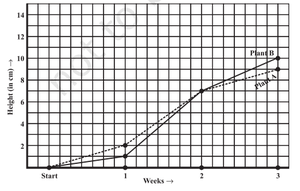(a) How high was Plant A after (i) 2 weeks (ii) 3 weeks?

Solution:

(i) Plant A was 7 cm’s high after 2 weeks.

(ii) Plant A was 9 cm’s high after 3 weeks.

(b) How high was Plant B after (i) 2 weeks (ii) 3 weeks?

Solution:

(i) Plant B was 7 cm’s high after 2 weeks.

(ii) Plant B was 10 cm’s high after 3 weeks.

(c) How much did Plant A grow during the 3rd week?

Solution:

Plant A’s height after 2 weeks was 7 cm’s and
Plant A’s height after 3 weeks was 9 cm’s
therefore, Plant A grew 2 cm’s in the 3rd week (which is 9 cm’s – 7 cm’s).

(d) How much did Plant B grow from the end of the 2nd week to the end of the 3rd week?

Solution:

Plant B’s height after 2 weeks was 7 cm’s and
Plant B’s height after 3 weeks was 10 cm’s
therefore, Plant B grew 3 cm’s from the end of the 2nd week to the end of the 3rd week (which is 10 cm’s – 7 cm’s).

(e) During which week did Plant A grow most?

Solution:

2nd week.

Explanation: Plant A grew 2 cm’s in 1st week, 5 cm’s in 2nd week, 2 cm’s in 3rd week. Therefore, Plant A grew most in 2nd week.

(f) During which week did Plant B grow least?

Solution:

1st week.

Explanation: Plant B grew 1 cm in 1st week, 6 cm’s in 2nd week, 3 cm’s in 3rd week. Therefore, Plant B grew Least in 1st week.

(g) Were the two plants of the same height during any week shown here? Specify.

Solution:

Yes, after 2 weeks both the plants are at the same height (which is 7 cm).

### Question 4: The following graph shows the temperature forecast and the actual temperature for each day of a week.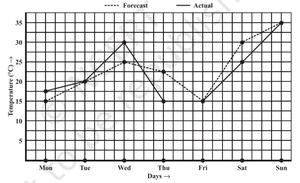(a) On which days was the forecast temperature the same as the actual temperature?

Solution:

On Tuesday, Friday, Sunday forecast temperature was the same as the actual temperature.

(b) What was the maximum forecast temperature during the week?

Solution:

35oC was the maximum forecast temperature during the week.

(c) What was the minimum actual temperature during the week?

Solution:

15oC was the minimum actual temperature during the week.

(d) On which day did the actual temperature differ the most from the forecast temperature?

Solution:

On Thursday, the difference between actual temperature and forecast temperature is 15oC and it is
the maximum among all the days.

### Question 5: Use the tables below to draw linear graphs.

(a) The number of days a hillside city received snow in different years.

Solution: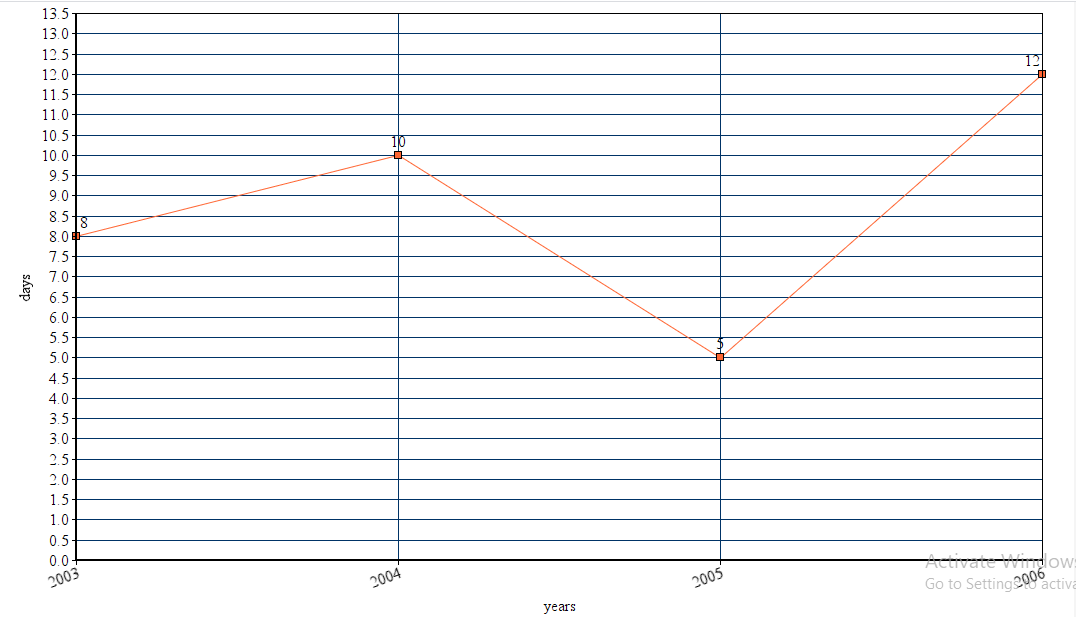(b) Population (in thousands) of men and women in a village in different years.

Solution: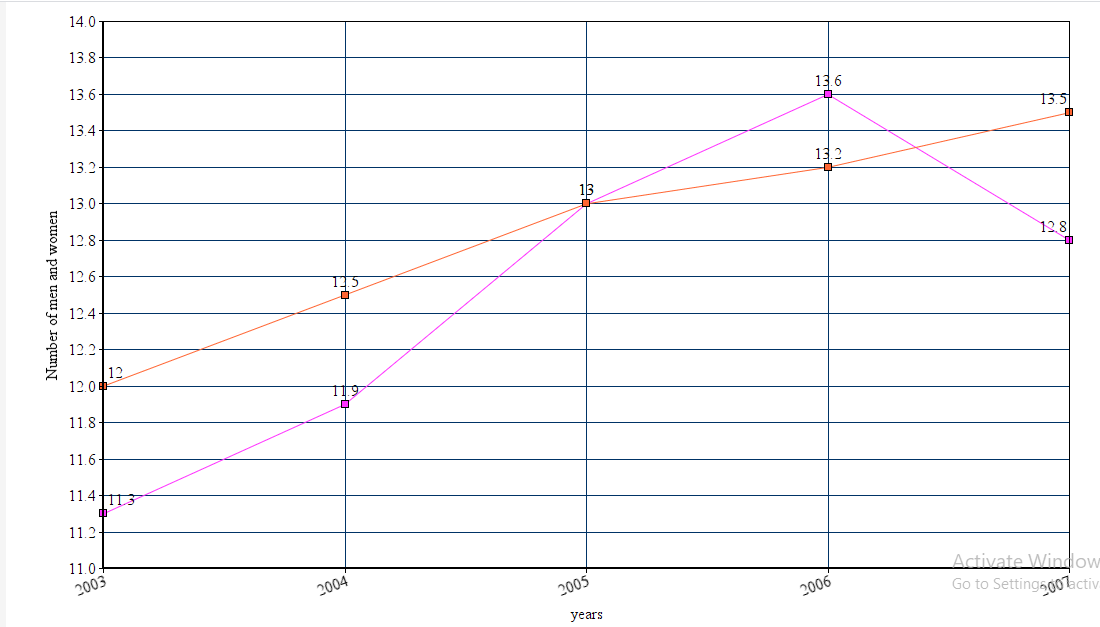### Question 6: A courier-person cycles from a town to a neighboring suburban area to deliver a parcel to a merchant. His distance from the town at different times is shown by the following graph.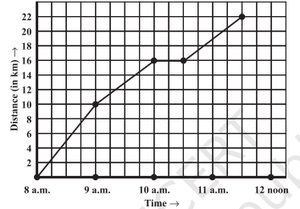(a) What is the scale taken for the time axis?

Solution:

4 Units = 1 hour

Therefore, 1 Unit = 1/4 hour

1 Unit = 15 mins.

(b) How much time did the person take for the travel?

Solution:

The person traveled from 8:00 a.m. to 11:30 a.m.
Therefore, the person took 3 hours and 30 mins to travel.

(c) How far is the place of the merchant from the town?

Solution:

The distance between the place of a merchant from the town is 22 km.

(d) Did the person stop on his way? Explain.

Solution:

Yes, the person stopped on his way.
In between 10:00 a.m. and 10:30 a.m., his distance from the town remained the same.

(e) During which period did he ride fastest?

Solution:

In between 8:00 a.m. and 9:00 a.m. (he traveled 10 km during this period and it is the maximum).

### Question 7: Can there be a time-temperature graph as follows? Justify your answer.

(i)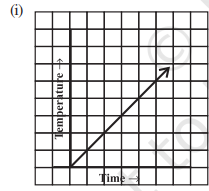Solution:

Yes, this graph shows the raise in temperature with respect to time.

(ii)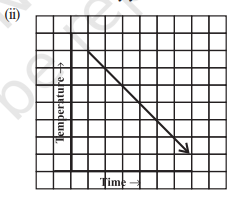Solution:

Yes, this graph shows the decrease in temperature with respect to time.

(iii)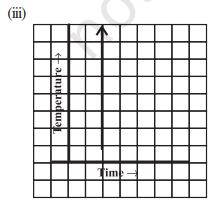Solution:

No, Temperature cannot have different values at a given time.

(iv)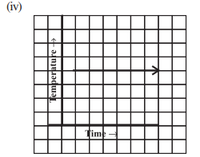Solution:

Yes, this graph says the temperature is constant with respect to time.

My Personal Notes arrow_drop_up